Home » A Step-by-Step Introduction to the Basic Object Detection Algorithms (Part 1)

# A Step-by-Step Introduction to the Basic Object Detection Algorithms (Part 1)

## Introduction

How much time have you spent looking for lost room keys in an untidy and messy house? It happens to the best of us and till date remains an incredibly frustrating experience. But what if a simple computer algorithm could locate your keys in a matter of milliseconds?

That is the power of object detection algorithms. While this was a simple example, the applications of object detection span multiple and diverse industries, from round-the-clock surveillance to real-time vehicle detection in smart cities. In short, these are powerful deep learning algorithms.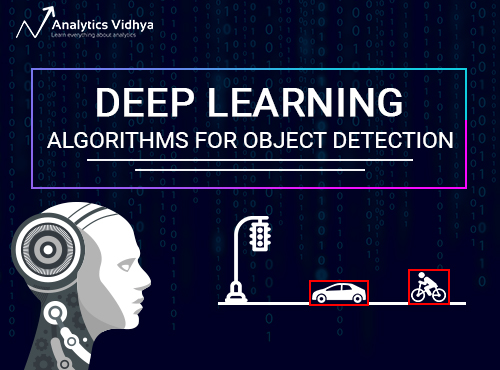In this article specifically, we will dive deeper and look at various algorithms that can be used for object detection. We will start with the algorithms belonging to RCNN family, i.e. RCNN, Fast RCNN and Faster RCNN. In the upcoming article of this series, we will cover more advanced algorithms like YOLO, SSD, etc.

I encourage you to go through this previous article on object detection, where we cover the basics of this wonderful technique and show you an implementation in Python using the ImageAI library.

Part 2 and Part 3 of this series are also published now. You can access them here:

Let’s get started!

1. A Simple Way of Solving an Object Detection Task (using Deep Learning)
2. Understanding Region-Based Convolutional Neural Networks
1. Intuition of RCNN
2. Problems with RCNN
3. Understanding Fast RCNN
1. Intuition of Fast RCNN
2. Problems with Fast RCNN
4. Understanding Faster RCNN
1. Intuition of Faster RCNN
2. Problems with Faster RCNN
5. Summary of the Algorithms covered

## 1. A Simple Way of Solving an Object Detection Task (using Deep Learning)

The below image is a popular example of illustrating how an object detection algorithm works. Each object in the image, from a person to a kite, have been located and identified with a certain level of precision.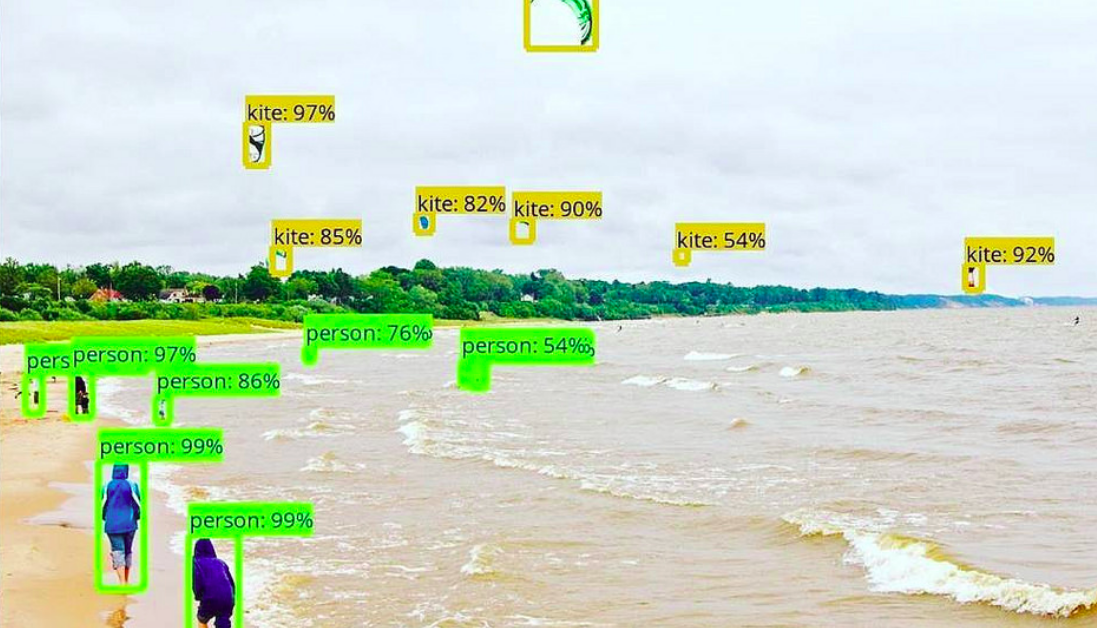Let’s start with the simplest deep learning approach, and a widely used one, for detecting objects in images – Convolutional Neural Networks or CNNs. If your understanding of CNNs is a little rusty, I recommend going through this article first.

But I’ll briefly summarize the inner workings of  a CNN for you. Take a look at the below image: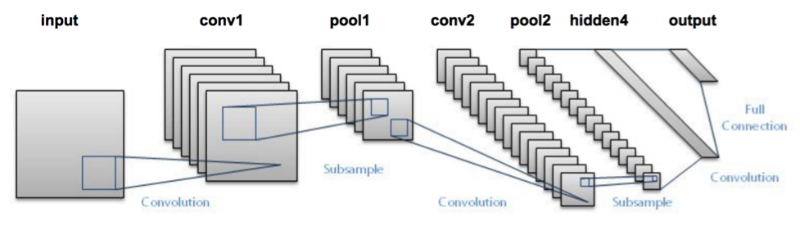We pass an image to the network, and it is then sent through various convolutions and pooling layers. Finally, we get the output in the form of the object’s class. Fairly straightforward, isn’t it?

For each input image, we get a corresponding class as an output. Can we use this technique to detect various objects in an image? Yes, we can! Let’s look at how we can solve a general object detection problem using a CNN.

1. First, we take an image as input: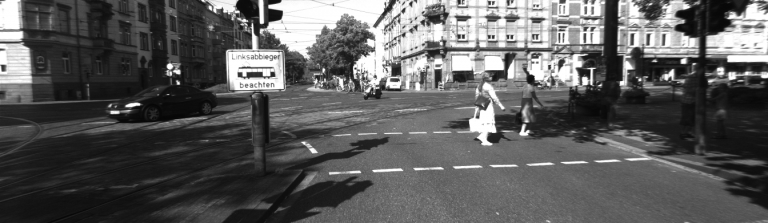2. Then we divide the image into various regions: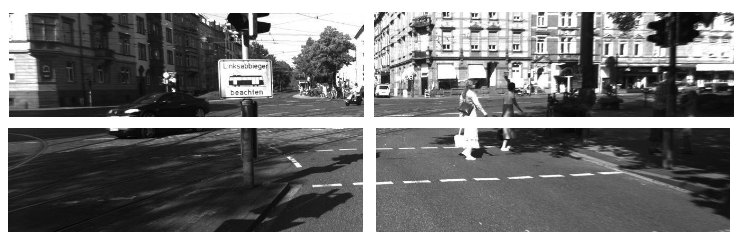3. We will then consider each region as a separate image.
4. Pass all these regions (images) to the CNN and classify them into various classes.
5. Once we have divided each region into its corresponding class, we can combine all these regions to get the original image with the detected objects: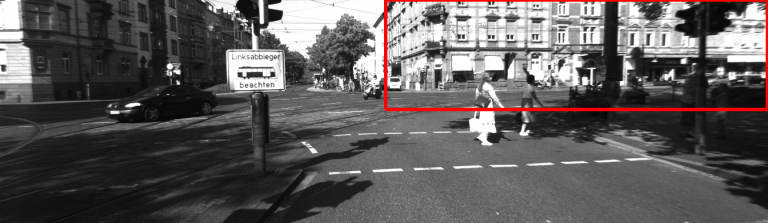The problem with using this approach is that the objects in the image can have different aspect ratios and spatial locations. For instance, in some cases the object might be covering most of the image, while in others the object might only be covering a small percentage of the image. The shapes of the objects might also be different (happens a lot in real-life use cases).

As a result of these factors, we would require a very large number of regions resulting in a huge amount of computational time. So to solve this problem and reduce the number of regions, we can use region-based CNN, which selects the regions using a proposal method. Let’s understand what this region-based CNN can do for us.

## 2. Understanding Region-Based Convolutional Neural Network

### 2.1 Intuition of RCNN

Instead of working on a massive number of regions, the RCNN algorithm proposes a bunch of boxes in the image and checks if any of these boxes contain any object. RCNN uses selective search to extract these boxes from an image (these boxes are called regions).

Let’s first understand what selective search is and how it identifies the different regions. There are basically four regions that form an object: varying scales, colors, textures, and enclosure. Selective search identifies these patterns in the image and based on that, proposes various regions. Here is a brief overview of how selective search works:

• It first takes an image as input:• Then, it generates initial sub-segmentations so that we have multiple regions from this image: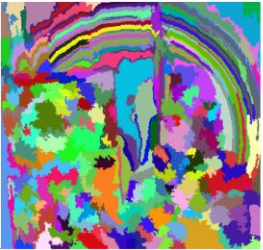• The technique then combines the similar regions to form a larger region (based on color similarity, texture similarity, size similarity, and shape compatibility):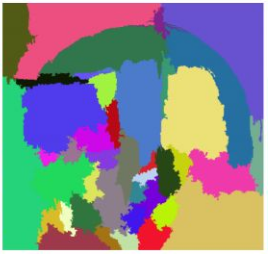• Finally, these regions then produce the final object locations (Region of Interest).

Below is a succint summary of the steps followed in RCNN to detect objects:

1. We first take a pre-trained convolutional neural network.
2. Then, this model is retrained. We train the last layer of the network based on the number of classes that need to be detected.
3. The third step is to get the Region of Interest for each image. We then reshape all these regions so that they can match the CNN input size.
4. After getting the regions, we train SVM to classify objects and background. For each class, we train one binary SVM.
5. Finally, we train a linear regression model to generate tighter bounding boxes for each identified object in the image.

You might get a better idea of the above steps with a visual example (Images for the example shown below are taken from this paper) . So let’s take one!

• First, an image is taken as an input: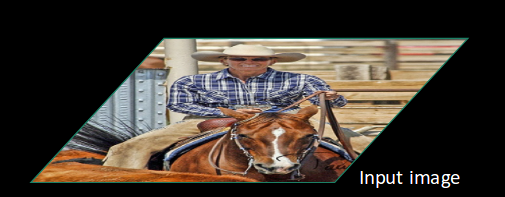• Then, we get the Regions of Interest (ROI) using some proposal method (for example, selective search as seen above):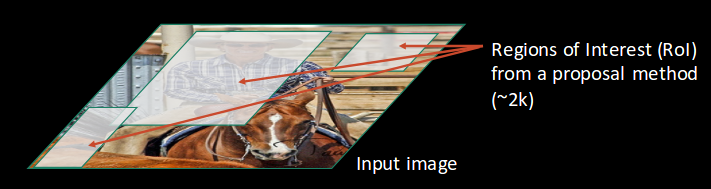• All these regions are then reshaped as per the input of the CNN, and each region is passed to the ConvNet: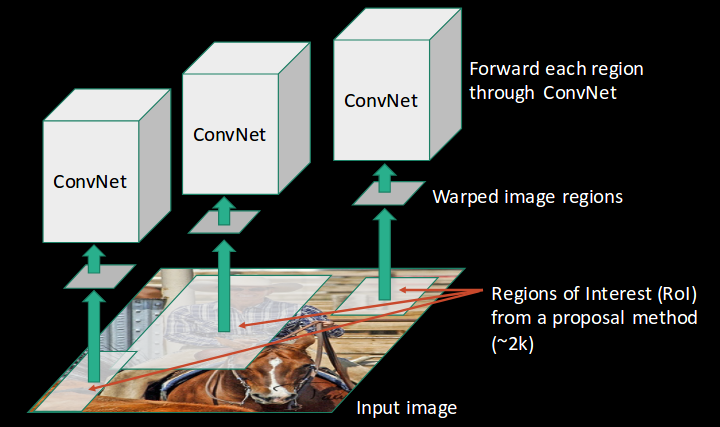• CNN then extracts features for each region and SVMs are used to divide these regions into different classes: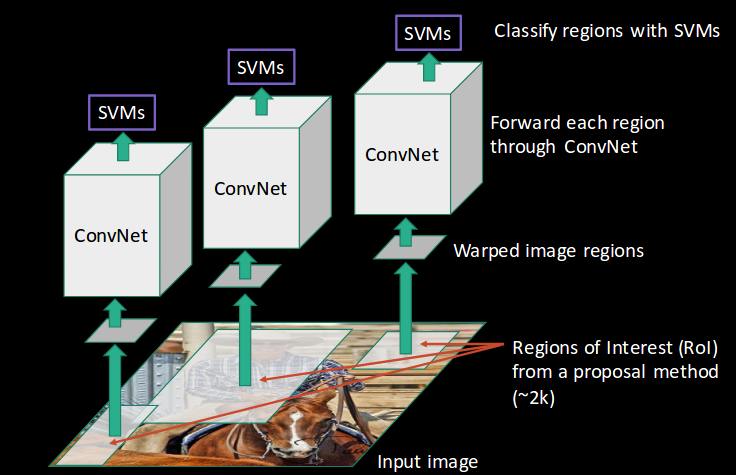• Finally, a bounding box regression (Bbox reg) is used to predict the bounding boxes for each identified region: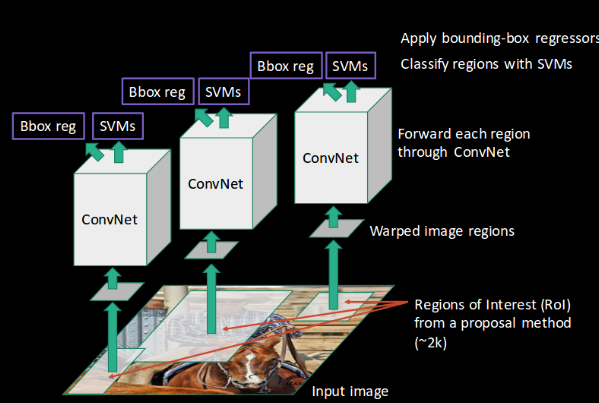And this, in a nutshell, is how an RCNN helps us to detect objects.

### 2.2 Problems with RCNN

So far, we’ve seen how RCNN can be helpful for object detection. But this technique comes with its own limitations. Training an RCNN model is expensive and slow thanks to the below steps:

• Extracting 2,000 regions for each image based on selective search
• Extracting features using CNN for every image region. Suppose we have N images, then the number of CNN features will be N*2,000
• The entire process of object detection using RCNN has three models:
1. CNN for feature extraction
2. Linear SVM classifier for identifying objects
3. Regression model for tightening the bounding boxes.

All these processes combine to make RCNN very slow. It takes around 40-50 seconds to make predictions for each new image, which essentially makes the model cumbersome and practically impossible to build when faced with a gigantic dataset.

Here’s the good news – we have another object detection technique which fixes most of the limitations we saw in RCNN.

## 3. Understanding Fast RCNN

### 3.1 Intuition of Fast RCNN

What else can we do to reduce the computation time a RCNN algorithm typically takes? Instead of running a CNN 2,000 times per image, we can run it just once per image and get all the regions of interest (regions containing some object).

Ross Girshick, the author of RCNN, came up with this idea of running the CNN just once per image and then finding a way to share that computation across the 2,000 regions. In Fast RCNN, we feed the input image to the CNN, which in turn generates the convolutional feature maps. Using these maps, the regions of proposals are extracted. We then use a RoI pooling layer to reshape all the proposed regions into a fixed size, so that it can be fed into a fully connected network.

Let’s break this down into steps to simplify the concept:

1. As with the earlier two techniques, we take an image as an input.
2. This image is passed to a ConvNet which in turns generates the Regions of Interest.
3. A RoI pooling layer is applied on all of these regions to reshape them as per the input of the ConvNet. Then, each region is passed on to a fully connected network.
4. A softmax layer is used on top of the fully connected network to output classes. Along with the softmax layer, a linear regression layer is also used parallely to output bounding box coordinates for predicted classes.

So, instead of using three different models (like in RCNN), Fast RCNN uses a single model which extracts features from the regions, divides them into different classes, and returns the boundary boxes for the identified classes simultaneously.

To break this down even further, I’ll visualize each step to add a practical angle to the explanation.

• We follow the now well-known step of taking an image as input:• This image is passed to a ConvNet which returns the region of interests accordingly: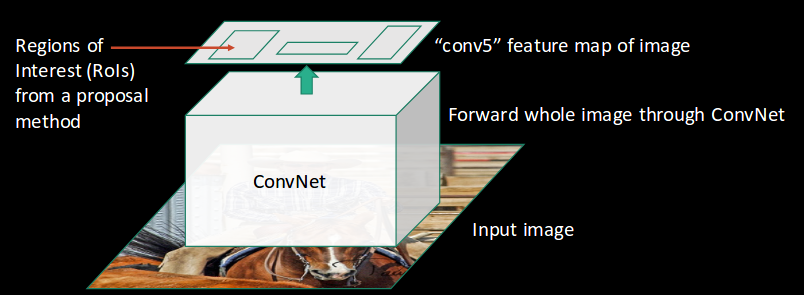• Then we apply the RoI pooling layer on the extracted regions of interest to make sure all the regions are of the same size: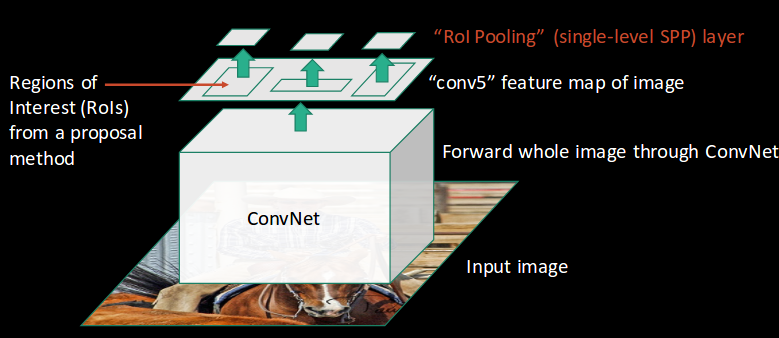• Finally, these regions are passed on to a fully connected network which classifies them, as well as returns the bounding boxes using softmax and linear regression layers simultaneously: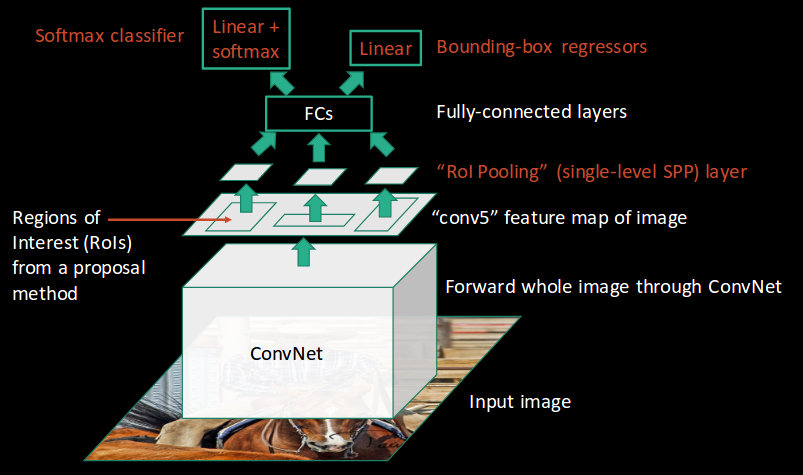This is how Fast RCNN resolves two major issues of RCNN, i.e., passing one instead of 2,000 regions per image to the ConvNet, and using one instead of three different models for extracting features, classification and generating bounding boxes.

### 3.2 Problems with Fast RCNN

But even Fast RCNN has certain problem areas. It also uses selective search as a proposal method to find the Regions of Interest, which is a slow and time consuming process. It takes around 2 seconds per image to detect objects, which is much better compared to RCNN. But when we consider large real-life datasets, then even a Fast RCNN doesn’t look so fast anymore.

But there’s yet another object detection algorithm that trump Fast RCNN. And something tells me you won’t be surprised by it’s name.

## 4. Understanding Faster RCNN

### 4.1. Intuition of Faster RCNN

Faster RCNN is the modified version of Fast RCNN. The major difference between them is that Fast RCNN uses selective search for generating Regions of Interest, while Faster RCNN uses “Region Proposal Network”, aka RPN. RPN takes image feature maps as an input and generates a set of object proposals, each with an objectness score as output.

The  below steps are typically followed in a Faster RCNN approach:

1. We take an image as input and pass it to the ConvNet which returns the feature map for that image.
2. Region proposal network is applied on these feature maps. This returns the object proposals along with their objectness score.
3. A RoI pooling layer is applied on these proposals to bring down all the proposals to the same size.
4. Finally, the proposals are passed to a fully connected layer which has a softmax layer and a linear regression layer at its top, to classify and output the bounding boxes for objects.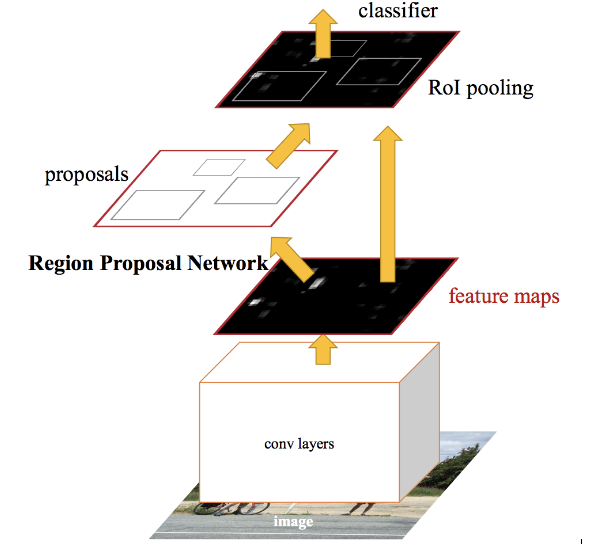Let me briefly explain how this Region Proposal Network (RPN) actually works.

To begin with, Faster RCNN takes the feature maps from CNN and passes them on to the Region Proposal Network. RPN uses a sliding window over these feature maps, and at each window, it generates k Anchor boxes of different shapes and sizes: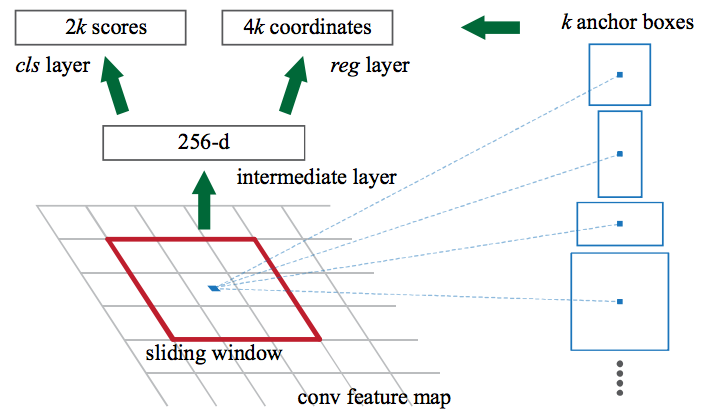Anchor boxes are fixed sized boundary boxes that are placed throughout the image and have different shapes and sizes. For each anchor, RPN predicts two things:

• The first is the probability that an anchor is an object (it does not consider which class the object belongs to)
• Second is the bounding box regressor for adjusting the anchors to better fit the object

We now have bounding boxes of different shapes and sizes which are passed on to the RoI pooling layer. Now it might be possible that after the RPN step, there are proposals with no classes assigned to them. We can take each proposal and crop it so that each proposal contains an object. This is what the RoI pooling layer does. It extracts fixed sized feature maps for each anchor: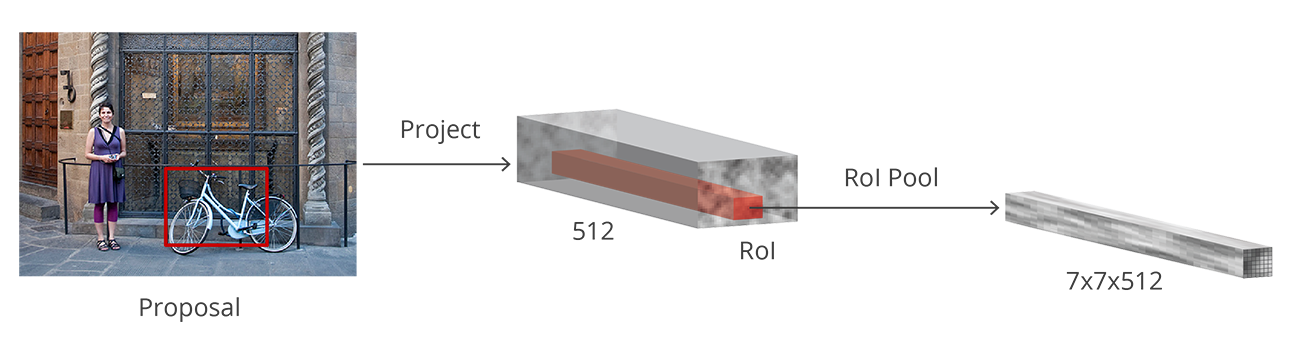Then these feature maps are passed to a fully connected layer which has a softmax and a linear regression layer. It finally classifies the object and predicts the bounding boxes for the identified objects.

### 4.2 Problems with Faster RCNN

All of the object detection algorithms we have discussed so far use regions to identify the objects. The network does not look at the complete image in one go, but focuses on parts of the image sequentially. This creates two complications:

• The algorithm requires many passes through a single image to extract all the objects
• As there are different systems working one after the other, the performance of the systems further ahead depends on how the previous systems performed

## 5. Summary of the Algorithms covered

The below table is a nice summary of all the algorithms we have covered in this article. I suggest keeping this handy next time you’re working on an object detection challenge!

 Algorithm Features Prediction time / image Limitations CNN Divides the image into multiple regions and then classify each region into various classes. – Needs a lot of regions to predict accurately and hence high computation time. RCNN Uses selective search to generate regions. Extracts around 2000 regions from each image. 40-50 seconds High computation time as each region is passed to the CNN separately also it uses three different model for making predictions. Fast RCNN Each image is passed only once to the CNN and feature maps are extracted. Selective search is used on these maps to generate predictions. Combines all the three models used in RCNN together. 2 seconds Selective search is slow and hence computation time is still high. Faster RCNN Replaces the selective search method with region proposal network which made the algorithm much faster. 0.2 seconds Object proposal takes time and as there are different systems working one after the other, the performance of systems depends on how the previous system has performed.

## End Notes

Object detection is a fascinating field, and is rightly seeing a ton of traction in commercial, as well as research applications. Thanks to advances in modern hardware and computational resources, breakthroughs in this space have been quick and ground-breaking.

This article is just the beginning of our object detection journey. In the next article (Part 2 and Part 3) of this series, we will encounter modern object detection algorithms such as YOLO and RetinaNet. So stay tuned!

I always appreciate any feedback or suggestions on my articles, so please feel free to connect with me in the comments section below.

You can also read this article on Analytics Vidhya's Android APP•Jacques GOUIMENOU says:

Very interesting paper. Good job!!!

•Pulkit Sharma says:

Thank You Jacques!

•Digambar Dewarde says:

Excellent Article..Really its helpfull for beginers..Thanks a lot..!!

•Pulkit Sharma says:

Hi Digambar,

•Shubham Jain says:

Hey Pulkit,

Interesting that you compiled so much information in a relatively very easy manner to grasp.
Thank you, Awesome job! 🙂

•Pulkit Sharma says:

Thank you Shubham!

•Arshad Kazi says:

Sir, I actually had one doubt that the time which you have mentioned for the algorithm execution are training in the summary is on which device, one a normal CPU or a GPU ? If it is a GPU, then what type of GPU. I am having a laptop with i5 processors and NVIDIA GetForce 940M series 2GB graphics card

•Pulkit Sharma says:

Any GPU will work. It will reduce the training time of the model. There will not be any effect on the model performance.

•Himakar says:

Hi Pulkit,

I am new to the neural network. but this seems to be very interesting.

•Pulkit Sharma says:

•Rahul Patel says:

Thanks for writing, it really helps me to get started in Object Detection.

In 2019, can you tell which one is best for object detection?

SSD: Single Shot MultiBox Detector
R-FCN
Faster RCNN
YOLO
Fast RCNN

•Pulkit Sharma says:

Hi Rahul,
It all depends on the type of problem and images that you are dealing with. Personally, I would start with YOLO as it is faster and performs equally well as compared to faster rcnn. But you can try different algorithms too depending on the problem at hand. If you want to understand how YOLO works and look at its implementation, you can refer to this blog.

•Sasi says:

Sir please link will be given to the starting of neural network…. Kind request

•Pulkit Sharma says:

Hi Sasi,

Can you please be more specific with your question? That would help me to clarify your doubt in a better way. Are you starting with Neural Network and want some references? or is it something else?

•panneer selvam says:

Nice article Pulkit Sharma. I am eager to see your part 2. Is it released by now ? . If so please share the link

•Pulkit Sharma says:

Hi,
Here are the part 2 and part 3 of this series of article.

•Bharatham says:

Nicely structured article Pulkit. Just wanted to ask something. Did you implement all three methods to compare the performance? If you have have could you please post the github link or something.

•Pulkit Sharma says:

Hi Bharatham,
I have implemented the Faster RCNN and have also written a blog on it. Have a look at the blog. I hope it will be helpful for you.

•Padmaja says:

Hi Pulkit, I m learning YOLO and your blog is really helpful for me. Please share your email id with me, I m a researcher n new to this area.

•Pulkit Sharma says: